# Tic Tac Toe - Subtracting Mixed Numbers

10 teachers like this lesson
Print Lesson

## Objective

SWBAT practice subtracting mixed number with like denominators through a tic tac toe game.

#### Big Idea

In this lesson students work with a partner to play a tic tac toe game in order to practice the skill of subtracting mixed numbers. Students can use visual fraction model and number strategies in order to subtract mixed numbers.

## Warm Up

7 minutes

Students will start today's lesson with a fluency assessment.  This assessment is from Monitoring Basic Skills Progress Second Edition: Basic Math Computation by Lynn S. Fuchs, Carol L. Hamlett, and Douglas Fuchs.

This is an assessment I have my students do each week and then graph their results.  It allows them to reflect on their learning of basic math facts, as well as using all four operations with whole numbers, and adding and subtracting unit fractions.  (It also happens to be the quietest time in my math classroom all week!!)

This is what my classroom looks like as students work on this assessment.

This is sample of a student's graph. You can also click here to hear my thoughts about this graph below.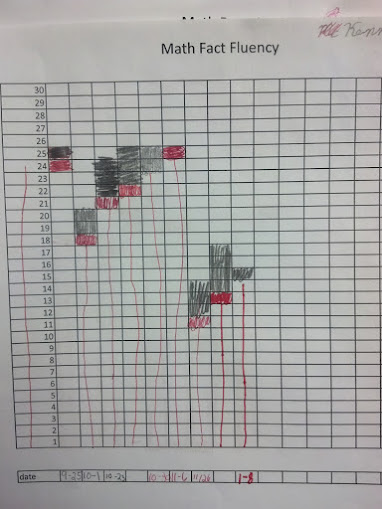Click hereto see an example of a typical fourth grade fluency decrease since students are completing the fourth grade fluency set that incorporates division, multiplication, addition, subtraction, and adding and subtracting fractions with like denominators. (At this point, students have not had many fraction lessons, thus very very students are able to complete the fraction problems)

I do not start my students with the fourth grade skills, but at this point in the year, all students are using the fourth grade set.. I chose to start them with the end of the third grade skills which covers addition, subtraction and multiplication and division of basic facts. I strongly believe in a balanced math approach, which is one reason why I also believe in common core standards.  By having a balance of building conceptual understanding, application of problems, and computational fluency, students can experience rigorous mathematics.  I want to make clear that this assessment ONLY measures basic math computation.  It is only one piece of students' knowledge. The assessments in this book, for each grade level, do not change in difficulty over the course of the year.  Therefore, a student's increase in score over the school year truly reflects improvement in the student's ability to work the math problems at that grade level.

## Concept development

30 minutes

In this lesson students start by reviewing strategies for adding mixed numbers with like denominators.   I give students this problem to solve:

4  7/8 + 3  5/8

Students work to solve the problem and then I call student volunteers to the document camera to show their solution and talk about what strategy they used.  As students solve the problem I circulate around the room and make mental note of the strategies students are using. Then when I ask volunteers to share their solutions, I purposely ask students who have different strategies in order to reach all learners.  Students share model strategies, adding the wholes and then the parts strategies, as well as turning both mixed numbers into improper fractions before adding.

Then, I review with students strategies for subtracting mixed numbers.  I direct students attention to the whiteboard where I have written three different strategies for subtracting mixed numbers. I ask students to just look and notice similarities and differences between the strategies for one minute.  During this one minute, students are silent and just observing.

This is a photograph of the board and three different mixed number subtraction strategies.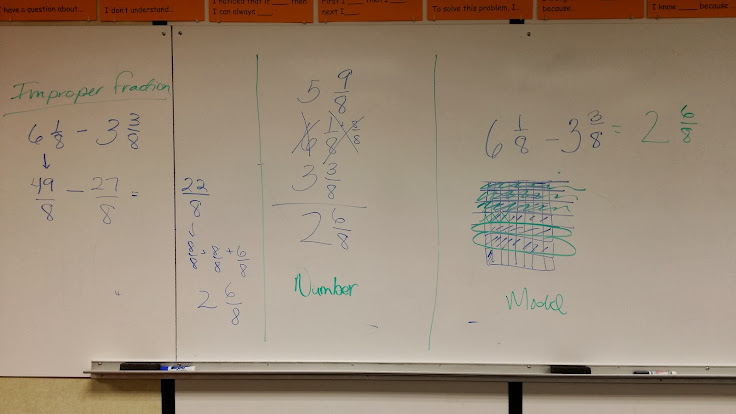After the one minute, I ask students what they notice that is the same about all of the strategies.  This helps students use Math Practice Standard 7, look for structure.  When students can observe patterns and similarities between strategies, they deepen their understanding preparing them for shortcut methods or algorithms.

Next, I direct students to fold a piece of paper into thirds, and then thirds the other way so they have a total of 9 rectangle sections or a tic tac toe board. I display 9 answers on the board and ask students to write one of the 9 answers in each square on their tic toe board. They write them in any order.  You can access the answers I used here - tic tac toe answers.docx

Once all students have their tic tac toe answers on their board, I then display three mixed number subtraction problems.  One of the reasons I chose to display three problems at once was for students who were early finishers. Students who finish the first problem do not have to wait for the entire class to finish the first problem, but can just continue with the second problem.  As students wait for all students to finish, I direct them to use another strategy to solve the first three problems.  This helps build their flexibility between strategies, thus deepening their understanding of subtracting mixed numbers.

Watch as this student explains a strategy he is using, or the strategy that his partner is using. You can see in this video that the student admits that he can not independently use the strategy that his partner is using. This was the perfect opportunity for me to step in and remind this student to ask his partner to slow down and explain more.  This student says he could have called for an "SOS" which I thought was very true. Whenever students work with partners, which is often in my classroom, it is imperative that I constantly circulate around the room and monitor students working together, keeping both on task, stepping in when necessary if one student isn't understanding his/her partner.

Another strategy I use to keep kids engaged and focused is to tell them a fun word to say when they do have a tic tac toe or three in a row.  For today's lesson, I asked students to yell out the word rutabaga when they have three in a row. They think this is silly, which it is, but it definitely a strategy that keeps them engaged.

In this photo you can see a student making her tic tac toe board.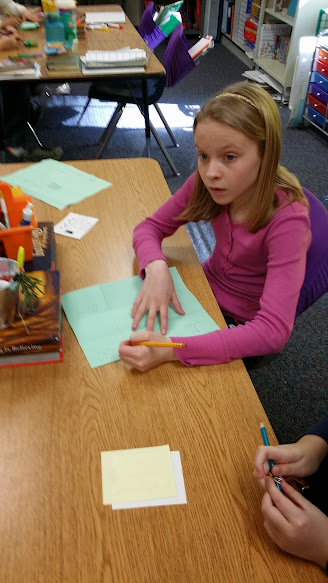You can see in this video a student explaining how to turn a mixed number into an improper fraction in order to subtract.  This is a very reluctant student who usually does not like to be video-ed at all.  While he didn't get an answer that was on his tac toe board (he should have), his partner is using a modeling strategy so I think this is a great benefit to both students. They can begin to analyze and evaluate how their strategies are the same and hopefully catch each other's mistakes.

## Student debrief - Wrap up

20 minutes

For this warm up I wanted to be able to gauge how well my students were with subtracting mixed numbers.  I direct all students to solve  6  4/6 - 3  5/6  as an exit ticket.

In this student's exit ticket you can see a modeling strategy.  The student is also able to see that 17/6 is another name for 2  5/6.  I had only a few students use this strategy.  I am still struggling with student abandoning modeling which is something I continue to work on.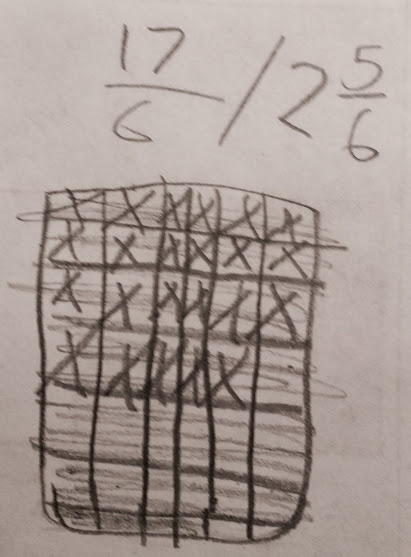You can see another strategy in this exit ticket photo of a student using an improper fraction method.  Many students were able to use this strategy and get a correct answer.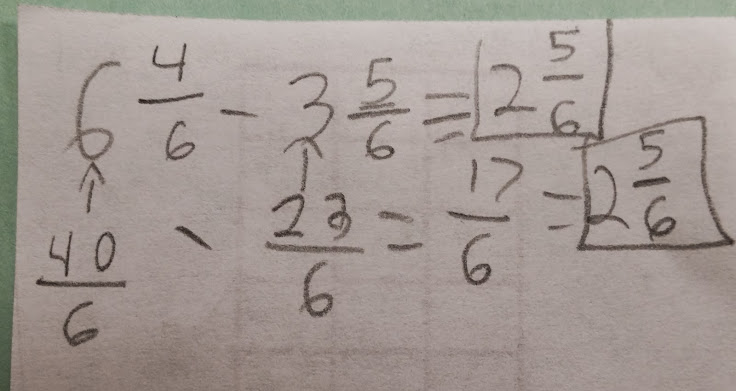You can see in this exit ticket that this student attempts converting the mixed numbers to improper fractions.  The student has failed to add in the fractional parts to the wholes when converting to mixed numbers, and therefore gets an incorrect answer.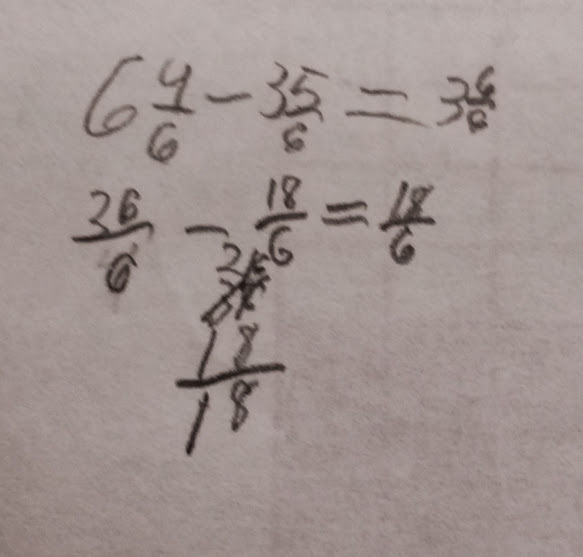In this photo of an exit ticket you can see the most common error my fourth graders made.  About 1/4 of my students attempted a number strategy and subtracted 4/6 from 5/6 instead of the other way around.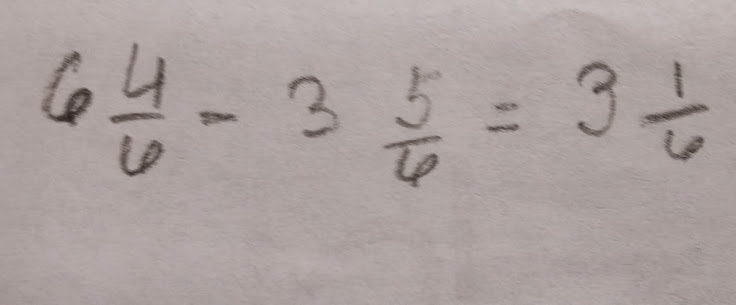I think it is important to note that for all of the above strategies, none of these strategies were taught to students.  These are strategies that they came up with simply by working with and modeling mixed numbers and fractions as in yesterday's lesson.